Equable Cylindrical Cone

EQUABLE CYLINDRICAL CONE

Balmoral Software

Solutions: 1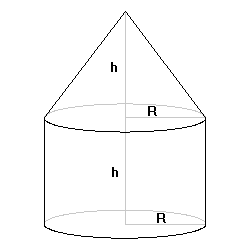Consider the "cylindrical cone" consisting of a cone of radius R and height h surmounting a cylinder of the same radius and height. There is one such polytope that is equable. The total volume of the cone + cylinder is V, where
V/π = (1/3)R2h + R2h = (4/3)R2h
The lateral surface area of the cone is Scone, whereThe lateral surface area of the cylinder is Scyl, where
 Scyl/π = 2Rh 
The surface area of the cylinder base is Sbase, where
 Sbase/π = R2 
The above four quantities are positive integers by convention. We have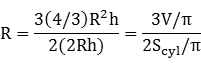so R is rational. By , R2 is an integer, so it follows that R is an integer.

The equability assumption is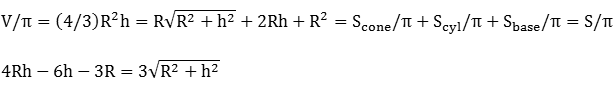Any equable solution will have a positive left side. Squaring both sides, we have
 (16R2 - 48R + 27)h = 24R2 - 36R 
If R = 1, then h = 12/5 and Scyl/π = 24/5 is not an integer. If R = 2, then h < 0. Therefore, R ≥ 3 and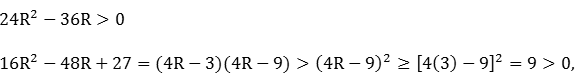so both the right side and the coefficient of h in  are strictly positive, and a rational solution for h is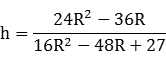From ,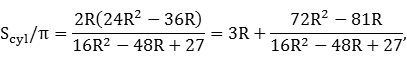so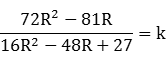for some integer k. We previously established that the denominator is positive, and the numerator is also positive since R ≥ 3, so k is positive. The preceding equation can be written as a quadratic in R:
 (16k - 72)R2 - (48k - 81)R + 27k = 0 
Since R is an integer, the discriminant of this quadratic must be a square:
576k2 + 6561 = m2 for some integer m

(m - 24k)(m + 24k) = 6561

The possibilities are summarized below:
 m - 24k m + 24k Sum = 2m m < k = [(m + 24k) - m]/24 1 < 6561 6562 3281 (6561 - 3281)/24 is not an integer 3 < 2187 2190 1095 (2187 - 1095)/24 is not an integer 9 < 729 738 369 (729 - 369)/24 = 15 27 < 243 270 135 (243 - 135)/24 is not an integer
The only solution is k = 15, and thus  can be written
168R2 - 639R + 405 = 0,
which has the sole integer solution R = 3. From , we have h = 4 and
V = 48 π

Scone = 15 π

Scyl = 24 π

Sbase = 9 π

The equable cylindrical cone is a solid of revolution of a right trapezoid with sides 3, 4, 5, 8 and diagonal 5, rotated around its longest side and having perimeter 20 and area 18.

### Non-equable case

It's interesting that without the equability restriction, the integer surface area sections have the property that
Scone2 = Sbase2 + (Scyl/2)2
Therefore, any Pythagorean triple a2 + b2 = c2, for which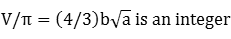generates the non-equable solution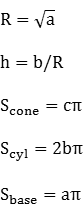For any Pythagorean triple, an alternate solution with a and b exchanged is possible as long as  is satisfied. Applying the limits on a and b, we have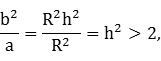so
h2 > 9/16 + 81/624 ≈ 0.6923
Any element of a Pythagorean triple is at least 3, so R2 = a ≥ 3 and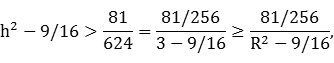which reduces to
V > Scone
Therefore, all solutions for which Scone ≤ M for some maximum M will include as a subset all solutions with V ≤ M. The 23 solutions having volume less than 2000 π are listed below:
RhSconeSbaseScylV/π
23/25468
341592448 *
4320162464
65/2393630120
340/341980160
415/2341660160
69/2453654216
1217/1214514434272
463/46516126336
68603696384
5126525120400
86806496512
677/68536154616
127/215014484672
635/211136210840
125156144120960
1015/21251001501000
680/3164363201280
815136642401280
912135812161296
1021/21451002101400
724175493361568
1291801442161728

*: Equable